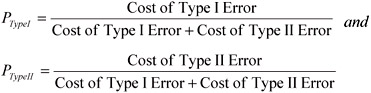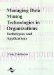# Integrating Type I and Type II Cost Preferences in Genetic-Algorithm-Based Classification Systems

 < Day Day Up >

We use the GA model described in "Pure Frontier Models" and incorporate Type I and Type II error costs. We name this model integrated cost preference based-GA model (ICPB-GA). In the ICPB-GA model, we first calculate the ratio (preference) of Type I and Type II error costs as follows,where PTypeI is the preference for minimization of Type I error and PTypeII is the preference for minimization of Type II error. The cost preferences can be directly incorporated into the fitness function of the genetic algorithm model. Since GAs use survival of the fittest strategy to evolve fit population members, we use the following fitness function to minimize priority-based Type I and Type II errors of misclassification.

Fitness = (Total Cases)-(PTypeI Total Type I Errors)-(PTypeII Total TypeII Errors)

The above fitness function is always positive since the total number of errors can never exceed total cases in the data set. Our model is different from the traditional classification model in which the fitness function maximizes correctly classified cases. The fitness function for the traditional model can be written as,

Fitness = (Total Cases)-(Total Type I Errors) - (Total Type II Errors)

The genetic learning procedure begins with a population of random strings and can be summarized as:

`   {      Randomly initialize coefficients of discriminant function ∈ [-1,1]      While (notterminating-condition){         evaluate-fitness of population members         perform tournament selection         With probability pcross         perform single-point crossover on two parents to get two new offsprings         With probability pmutate         perform mutation on a offspring         Replace parents with offsprings if offsprings have higher fitness        }        } `

The values of population members for the classification model for ICPB-GA is restricted between -1 and +1 to improve the speed and solution accuracy.

 < Day Day Up >Managing Data Mining Technologies in Organizations: Techniques and Applications
ISBN: 1591400570
EAN: 2147483647
Year: 2003
Pages: 174